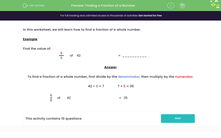# Find a Fraction of a Number

In this worksheet, students will find a fraction of a whole numberKey stage:  KS 3

Curriculum topic:   Number

Curriculum subtopic:   Use Four Operations for All Numbers

Popular topics:   Numbers worksheets

Difficulty level:#### Worksheet Overview

In this activity, we will learn how to find a fraction of a whole number.

Example

Find the value of:

5/6 of 42

To find a fraction of a whole number, first divide by the denominator, then multiply by the numerator.

42 ÷ 6 = 7               7 × 5 = 35

5/6 of 42 = 35

Let's have a go at some questions now.

### What is EdPlace?

We're your National Curriculum aligned online education content provider helping each child succeed in English, maths and science from year 1 to GCSE. With an EdPlace account you’ll be able to track and measure progress, helping each child achieve their best. We build confidence and attainment by personalising each child’s learning at a level that suits them.

Get started